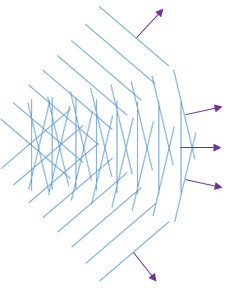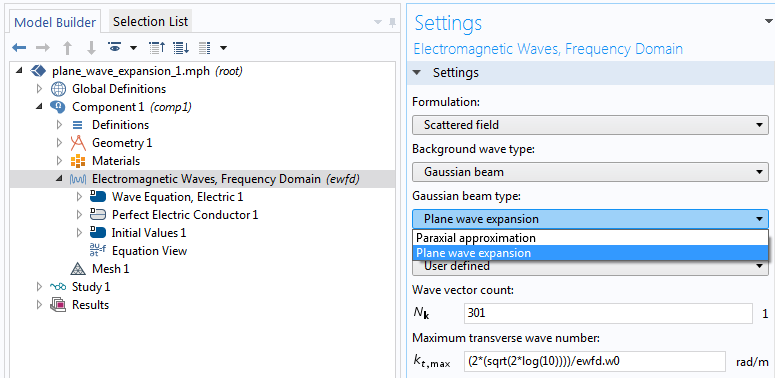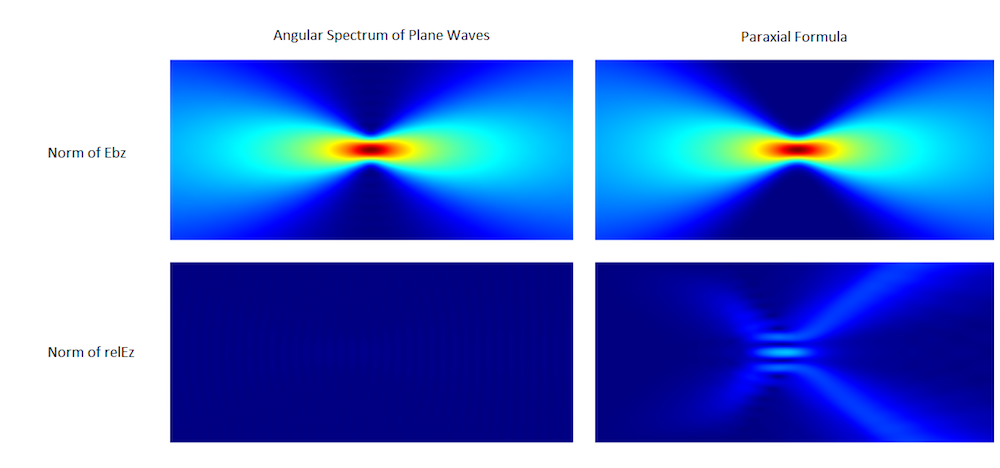# The Nonparaxial Gaussian Beam Formula for Simulating Wave Optics

June 26, 2018

In a previous blog post, we discussed the paraxial Gaussian beam formula. Today, we’ll talk about a more accurate formulation for Gaussian beams, available as of version 5.3a of the COMSOL® software. This formulation based on a plane wave expansion can handle nonparaxial Gaussian beams more accurately than the conventional paraxial formulation.

### Paraxiality of Gaussian Beams

The well-known Gaussian beam formula is only valid for paraxial Gaussian beams. Paraxial means that the beam mainly propagates along the optical axis. There are several papers that talk about paraxiality in a quantitative sense (see Ref. 1).

Roughly speaking, if the beam waist size is near the wavelength, the beam propagates at a higher angle to a focus. Therefore, the paraxiality assumption breaks down and the formulation is no longer accurate. To alleviate this problem and to provide you with a more general and accurate formulation for general Gaussian beams, we introduced a nonpariaxial Gaussian beam formulation. In the user interface this is referred to as Plane wave expansion.

The method is based on the angular spectrum of plane waves (Ref. 2) and is sometimes referred to as the angular spectrum method (Ref. 3).

### Angular Spectrum of Plane Waves

Let’s briefly review the paraxial Gaussian beam formula in 2D (for the sake of better visuals and understanding).

We start from Maxwell’s equations assuming time-harmonic fields, from which we get the following Helmholtz’s equation for the out-of-plane electric field with the wavelength \lambda for our choice of polarization:

\left ( \frac{\partial^2}{\partial x^2} +\frac{\partial^2}{\partial y^2} + k^2 \right ) E_z = 0,

where k=2 \pi/\lambda.

The angular spectrum of plane waves is based on the following simple fact: an arbitrary field that satisfies the above Helmholtz equation can be expressed as the following plane wave expansion:

E_z(x,y) = \int_{k_x^2+k_y^2=k^2} A(k_y)e^{i(k_x x +k_y y)} dk_y,

where A(k_y) is an arbitrary function.

The integration path is a circle of radius k for real k_x and k_y. (For complex k_x and k_y, the integration domain extends to a complex plane.) The function A(k_y) is called the angular spectrum function. One can prove that this E_z satisfies Helmholtz’s equation by direct substitution.

Now that we know that this formulation always gives exact solutions to Helmholtz’s equation, let’s try to understand it visually. From the constraint, k_x^2+k_y^2=k^2, we can set k_x=k cos(\varphi) and k_y=k sin(\varphi) and rewrite the above equation as:

E_z(x,y) = \int_{-\pi/2}^{\pi/2} A(\varphi)e^{ik(x \cos \varphi +y \sin \varphi)}d \varphi.

The meaning of the above formula is that it constructs a wave as a sum, or integral, consisting of many waves propagating in various directions, all with the same wave number k. This is shown in the following figure.Visualization of the angular spectrum of plane waves.

Back to k_y representation, when actually solving a problem using this formula, all you have to do is find the angular spectrum function A(k_y) that satisfies the boundary conditions. By assuming that the profile of the transverse field (perpendicular to the propagating direction, i.e., optical axis) is also a Gaussian shape (see Ref. 4), one can derive that A(k_y) = \exp(-k_y^2 / w_0^2) , where w_0 is the spectrum width.

By some more mathematical manipulations, we get a relationship between the spectrum width w_0 and the beam waist radius. For example, for a slow Gaussian beam, the angular spectrum is narrow. A plane wave, on the other hand, is the extreme case where the angular spectrum function is a delta function. For a fast Gaussian beam, the angular spectrum is wider, and vice versa.

This was a quick summary of the underlying theory for nonparaxial Gaussian beams. To recap what we have shown so far, let’s rewrite the formula once more by using polar coordinates, x=r \cos \theta, \ y = r \sin \theta:

E_z(r,\theta) = \int_{-\pi/2}^{\pi/2} A(\varphi) e^{ikr \cos (\theta-\varphi)}d \varphi.

This is the formulation that Born and Wolf (Ref. 2) use in their book.

The 3D formula is more complicated and looks different due to polarization, but the basic idea is the same as seen in the references mentioned above. It can also look different depending on whether or not you consider evanescent waves. The Plane Wave Expansion method used in the Wave Optics Module and the RF Module, although based on the angular spectrum theory, is adapted for numerical computations.

### Plane Wave Expansion: Settings and Results

Let’s compare the new feature, Plane wave expansion, with the previously available feature, Paraxial approximation. The Settings window covering both methods is shown below.The Plane Wave Expansion feature settings.

With the new feature, you have two options if the Automatic setting doesn’t give you a satisfactory approximation:

1. Wave vector count
2. Maximum transverse wave number

The first option determines the number of discretization levels, depending on how fine you want to represent the Gaussian beam. The more plane waves, the finer it gets. The second option is related to the integral bound in the previous equation; i.e., -\pi/2 \le \varphi \le \pi/2. This integral bound can be the maximum \pi/2 for the smallest possible spot size and can be more shallow for slower beams, depending on how fast the Gaussian beam is. You need more angled plane waves with a larger transverse wave number to represent faster (more focused) beams.

The following results compare the two formulas for the case where the spot radius is \lambda/2, which is considerably nonparaxial. As in the previous blog post, the simulation is done with the Scattered Field formulation and the domain is surrounded by a perfectly matched layer (PML). This way, the scattered field represents the error from the exact Helmholtz solution.

The left images below show the new feature, while the images on the right show the paraxial approximation. The top images show the norm of the computed Gaussian beam background field, ewfd.Ebz, while the bottom images show the scattered field norm,  ewfd.relEz, which represents the error from the exact Helmholtz solution. Obviously, the error from the Helmholtz solution is greatly reduced in the nonparaxial method.Comparison between the angular spectrum of plane waves and the paraxial formula.

### Concluding Remarks

We have discussed the theory and results for an approximation method for nonparaxial Gaussian beams using the new plane wave expansion option. Remember that this formulation is extremely accurate, but is still an approximation under assumptions. First, we have made an assumption for the field shape in the focal plane. Second, we assume that the evanescent field is zero. If you are interested in the field coupling to some nanostructure near the focal region in a fast Gaussian beam, you may need to calculate the evanescent field.

### Next Step

Learn more about the formulations and features available for modeling optically large problems in the COMSOL® software by clicking the button below:

Note: This functionality can also be found in the RF Module.

### References

1. P. Vaveliuk, “Limits of the paraxial approximation in laser beams”, Optics Letters, vol. 32, no. 8, 2007.
2. M. Born and E. Wolf, Principles of Optics, ed. 7, Cambridge University Press, 1999.
3. J. W. Goodman, Fourier Optics.
4. G. P. Agrawal and M. Lax, “Free-space wave propagation beyond the paraxial approximation”, Phys. Rev. a. 27, pp. 1693–1695, 1983.

#### Comentários (15)

##### Faça um Comentário
Carregando...##### Mohammed Mohammed
July 17, 2018

Dear Yosuke Mizuyama
Very interested topic. I have one question, please.
Why lambda is equal to 500nm and used in COMSOL as the default value for the calculation of frequency (f=c_const/500[nm])?##### Ryan Freeman
February 25, 2019

Has anyone been able use this method to create a gaussian beam that is focused to the diffraction limit?

I am currently trying to play around with the settings to create a tight focused beam, but the width of the resulting beam is around 5-10x too large.

Is there any more documentation on this method or new examples?

Thanks,
-Ryan##### Yosuke Mizuyama
February 25, 2019

Dear Ryan,

Thank you for reading my blog. If you didn’t get a focused beam, please try to increase the transverse wave number as I wrote in the post. Otherwise, please send your model to support@comsol.com.
Thank you!

Best regards,
Yosuke##### Michael Thomas
February 25, 2019

Hello Yosuke,

I have same problem.

I assume 2Pi/lambda is the maximal transverse wave number supported and correspond to maximal focusing.

Could problem maybe be trying to focus beam onto thin film (30nm)?

Excellent Blog Post Yosuke. Excited for new feature.

-Michael##### Yosuke Mizuyama
February 25, 2019

Dear Michael,
In the meantime, I will look into the functionality.
Best regards,
Yosuke##### Yosuke Mizuyama
February 27, 2019

Dear Ryan and Michael,
I simulated a half lambda waist beam. I think it’s good to show my observation for one parameter case here. This is almost close to the diffraction limited beam size. For such a high non-paraxiality, you need to modify the default settings. If you change “Wave vector distribution type” to “User defined”, you will see the two parameters, “Wave vector count”, Nk, and “Max transverse wave number”, kt,max. Please read the blog content for more details. I chose Nk=401 and kt,max=ewfd.k0/2 for getting a smooth result. The max transverse wave number does not necessarily need to be the highest, i.e., ewfd.k0, for diffraction limited beams because the contribution from such high angles is very small even for near diffraction limited beams. If you choose kt,max to be ewfd.k0, then Nk is not enough. You need to increase Nk to keep the same discretization level.
So far, you need this kind of consideration for really highly non-paraxial beams.
I hope this information is helpful.
Best regards,
Yosuke##### yuliang cheng
January 5, 2020

Dear Yosuke Mizuyama,
I want to let the gaussian beam incident on the metal film, and make the waist of the gaussian beam fall on the metal film, how should I set this?The gaussian beam is incident from the air medium to the surface of the metal medium.I can’t use your method to achieve the purpose, I want to consult you.Do I need to set the incident electromagnetic field formula?Can you give me a reference formula?
Thanks,
-Cheng##### Yosuke Mizuyama
January 6, 2020 COMSOL Employee

Dear Yuliang,

Thank you for reading my blog.
I think you can use the formula given in this blog post (the second equation).
Put your metal surface at x=0.
Set your excitation on the left input boundary, say x=-x0.
Calculate the excitation field based on the formula by substituting x=-x0 as a function of y.
You should be able to excite a non-paraxial Gaussian beam boundary field and to focus it on the metal surface placed at x=0.
BUT PLEASE read this blog again a couple of days later. I found a mistake in the second formula.
I will correct it now. It make take a couple days to update the page.
Thanks for making me read it again and find the error!

Best regards,
Yosuke##### Yosuke Mizuyama
January 6, 2020 COMSOL Employee

Dear Yuliang,

Now the correction has been made and the page is live.
The angular spectrum function A(ky) is exp(-ky^2/w0^2), where w0 is a constant that represents how fast the beam is.
Good luck!

Best regards,

Yosuke##### 妙 彭
April 24, 2020

Hi, Dr. Yosuke
I am sorry to bother you again.
I used the ” Plane wave expansion” approach to set the tightly focused gaussian beam, but when I increased the wave vector count to 100, an error occurred and the log file showed “java.lang.StackOverflowError”. I have no idea about this, can you help me ?
Best wishes
Sincerely,
Miao Peng##### Yosuke Mizuyama
April 24, 2020 COMSOL Employee

Hi Miao,
That doesn’t happen to me.
Please send the model file to support@comsol.com.
Best regards,
YosukeMay 26, 2020

Dear Yosuke Mizuyama,

is it possible to define a port that the focused beam starts from that?
when I used this method to simulate the propagation of highly focused gaussian beam in water, I found out by increasing the propagation length, the focus size start increasing, Why it happens? I think by default the beam starts from vaccuum environment and comes to defined medium, right?
I want the wave propagates from a glass medium to water medium. how can I change the initial medium of the wave?
Sincerely,##### Yosuke Mizuyama
May 27, 2020 COMSOL Employee

Thank you for reading my blog.
The port feature is supposed to be used for the transverse eigenmode fields.
So the Gaussian beam isn’t a right one for the port.
The material property is included in the wave vector as k = n*k0 in the formulation.
Best regards,
Yosuke
Instead, you can implement this in the Scattering Boundary Condition or Matched Boundary Condition as a user defined field.June 9, 2020

Thanks for your response Dear Yosuke Mizuyama,

Do you know how I can relate between beam radius and NA of objective lens?

Sincerely,